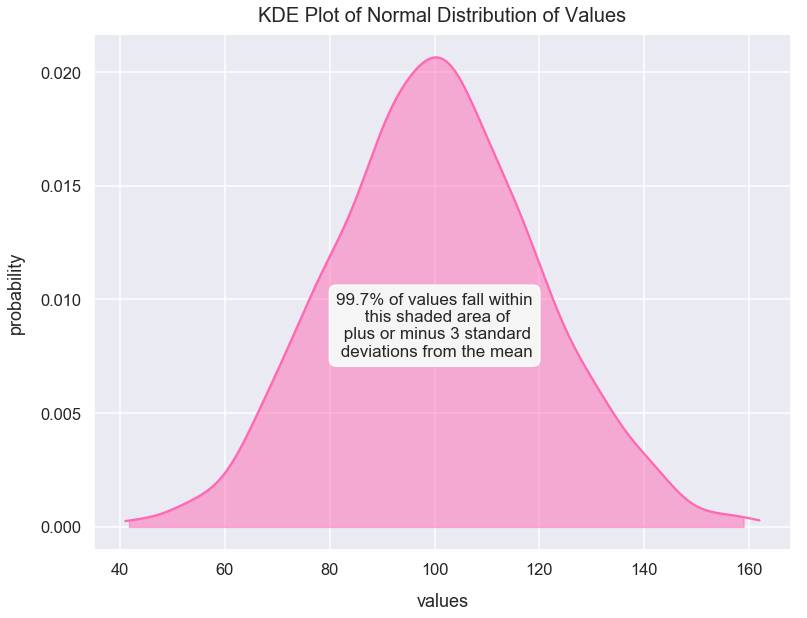Math Distributions Article

# Normal Distribution

A normal distribution has values in which the data has no bias left or right from its central value.

Many sets of data closely follow a normal distribution including:

• heights of people

### Import Modules¶

In :
import seaborn as sns
import numpy as np
import scipy
import warnings
import matplotlib.pyplot as plt
% matplotlib inline


I turn warnings off in this post because of an issue in Scipy that will be fixed in a later version.

In :
warnings.filterwarnings('ignore')


Visualization styling code

In :
sns.set(rc={'figure.figsize':(12.5, 9.5)})
sns.set_context('talk')


### Example 1: Build a Normal Distribution¶

Using the numpy package's random module, we can call the normal() method to create a list of values with a normal distribution by setting the following arguments:

• loc as the mean of the distribution
• scale as the standard deviation of the distribution
• size as number of samples
In :
np.random.seed(4) # seed random number generator with fixed value so we always get same values below
normal_distr_values = list(np.random.normal(loc=100, scale=20, size=1300))

In :
normal_distr_values = [int(value) for value in normal_distr_values]


Below is a plot of a histogram of these values that resemble a normal distribution.

In :
sns.distplot(normal_distr_values, kde=False, color='hotpink')
plt.title("Normal Distribution of Values", fontsize=20, y=1.012)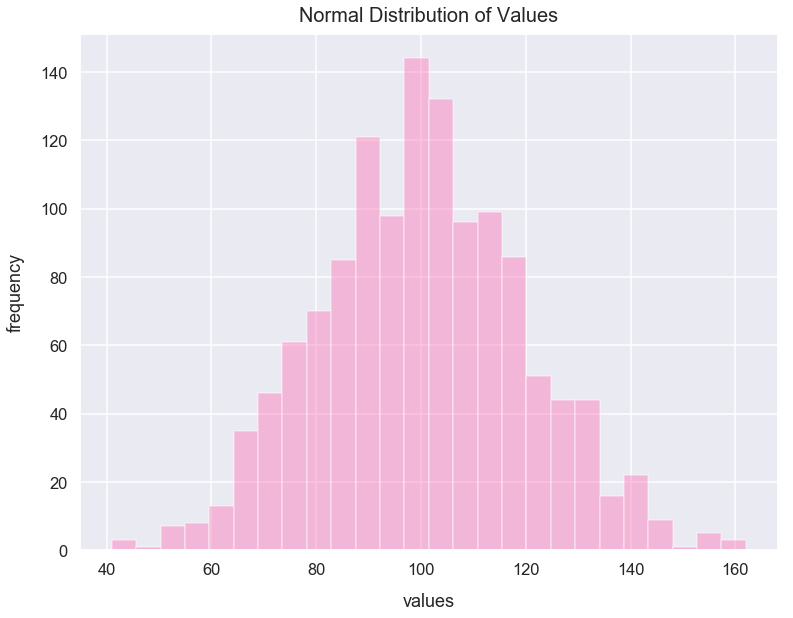This curve above is often called a "bell curve" since it looks like a bell seen below.When we say the data is "normally distributed", the normal distribution should have the following characteristics:

• mean = median = mode
• roughly 50% values less than the mean and 50% greater than the mean

From a visual standpoint, it looks like our distribution above has symmetry around the center.

Let's check the mean, median and mode values are roughly equal to one another.

##### Mean of Values¶
In :
mean = round(np.mean(normal_distr_values), 3)
mean

Out:
100.325
##### Median of Values¶
In :
median = round(np.median(normal_distr_values), 3)
median

Out:
100.0
##### Mode of Values¶
In :
mode = scipy.stats.mode(normal_distr_values)
mode

Out:
ModeResult(mode=array(), count=array())

100 equals 100 which is very close to 101. So, it seems in this dataset that the mean, median and mode are the same values.

##### Verify 50% of values less than mean and 50% above mean¶
In :
count_values_above_mean = 0

for value in normal_distr_values:
if value>=mean:
count_values_above_mean+=1

count_values_above_mean

Out:
643
In :
count_values_below_mean = len(normal_distr_values) - count_values_above_mean
count_values_below_mean

Out:
657

643 values are above the mean and 657 values are below the mean. This roughly fits our criteria to help prove that this is a normal distribution.

### Standard Deviations with a Normal Distribution¶

For the normal_distr_values we plotted above in a histogram. I can also plot it in KDE form which you can simply think of as a line of best fit to smooth out the histogram. You can learn more about KDE plots in Python through this great Jake Vanderplas article.

Below is our result.

In :
sns.distplot(normal_distr_values, rug=False, hist=False, color='hotpink')
plt.title("KDE Plot of Normal Distribution of Values", fontsize=20, y=1.012)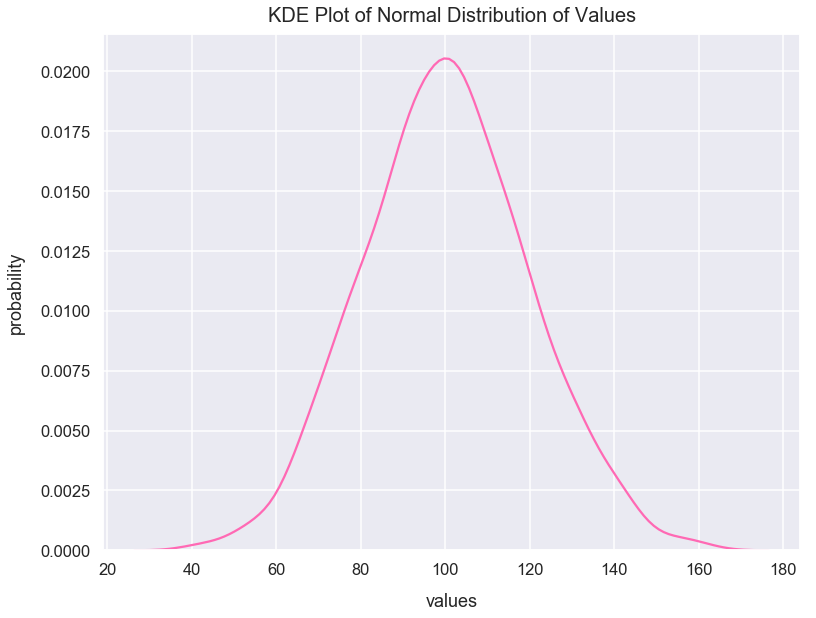Let's calculate the values for one, two and three positive standard deviations from the mean of normal_distr_values, and one, two and three negative standard deviations from the mean of normal_distr_values.

In :
plus_one_std_dev = np.mean(normal_distr_values) + np.std(normal_distr_values)
minus_one_std_dev = np.mean(normal_distr_values) - np.std(normal_distr_values)

plus_two_std_dev = np.mean(normal_distr_values) + 2*np.std(normal_distr_values)
minus_two_std_dev = np.mean(normal_distr_values) - 2*np.std(normal_distr_values)

plus_three_std_dev = np.mean(normal_distr_values) + 3*np.std(normal_distr_values)
minus_three_std_dev = np.mean(normal_distr_values) - 3*np.std(normal_distr_values)


Since we have a normal distribution, the following logic on standard deviations applies to this dataset.

Here's a Stack Overflow article with good code on how to use shading under plots in Matplotlib.

In :
kde = scipy.stats.gaussian_kde(normal_distr_values)
pos = np.linspace(min(normal_distr_values), max(normal_distr_values), 50000)
plt.plot(pos, kde(pos), color='hotpink')
plt.text(x=100.5, y=.0085, horizontalalignment='center', fontsize=17,
s="68% of values fall within\nthis shaded area of\nplus or minus 1 standard\ndeviation from the mean",
plt.title("KDE Plot of Normal Distribution of Values", fontsize=20, y=1.012)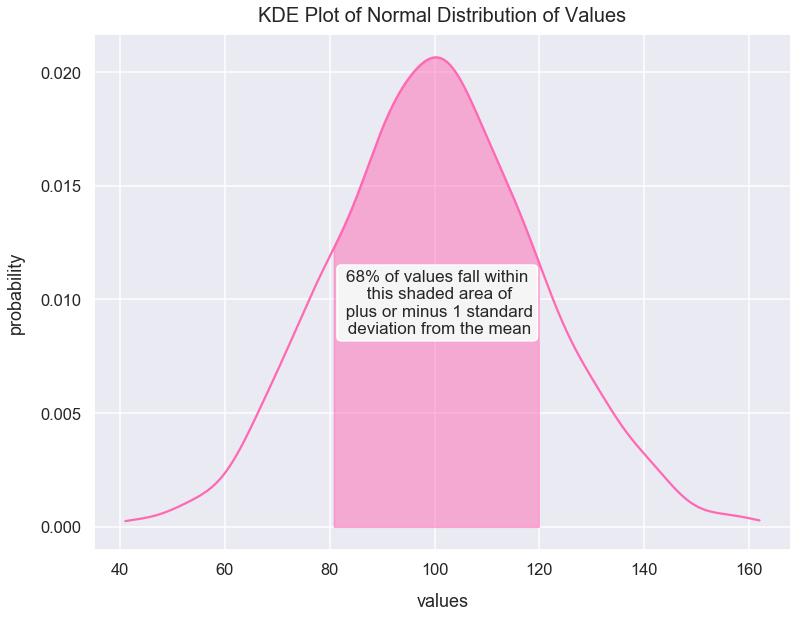In :
kde = scipy.stats.gaussian_kde(normal_distr_values)
pos = np.linspace(min(normal_distr_values), max(normal_distr_values), 5000)
plt.plot(pos, kde(pos), color='hotpink')
plt.text(x=100, y=.0075, horizontalalignment='center', fontsize=17,
s="95% of values fall within\nthis shaded area of\n plus or minus 2 standard\ndeviations from the mean",
plt.title("KDE Plot of Normal Distribution of Values", fontsize=20, y=1.012)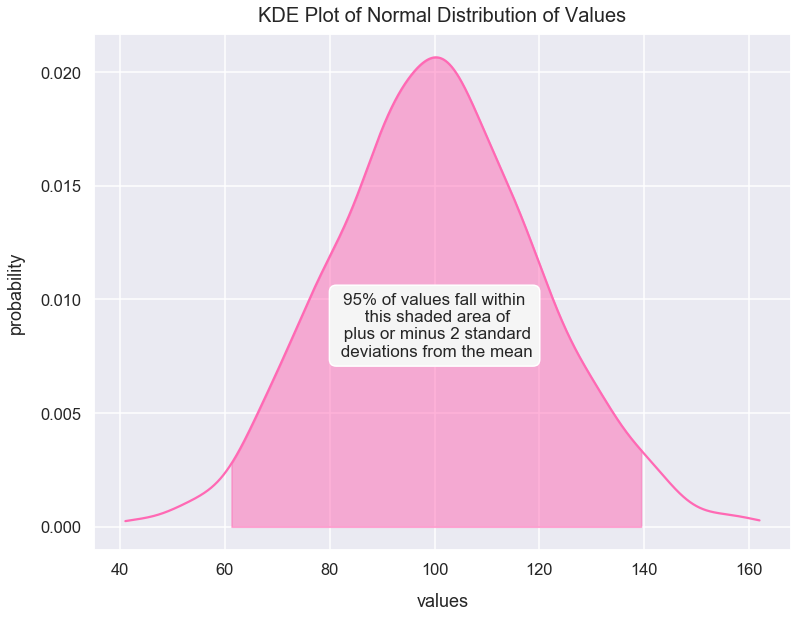In :
kde = scipy.stats.gaussian_kde(normal_distr_values)
pos = np.linspace(min(normal_distr_values), max(normal_distr_values), 5000)
plt.plot(pos, kde(pos), color='hotpink')